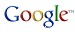VB Migration Partner

[PRB] The .NET application doesn’t compile because of a “Number of indices exceeds the number of dimensions of the indexed array” compilation error

In most cases, VB Migration Partner can infer the rank (i.e. the number of dimensions) of an array that isn't initialized in its Dim statement, as in this VB6 code:

```        Dim arr() As Integer
' ...
ReDim arr(10, 20) As Integer```

which is correctly migrated as follows:

```        ' VB.NET
Dim arr(,) As Integer
' ...
ReDim arr(10, 20) As Integer```
```        // C#
int[,] arr = null;
' ...
arr = new int[11,21];```

In a few cases, however, it isn't possible for VB Migration Partner to infer the array rank. This happens, for example, for functions that return an array. Consider the following VB6 code:

```        Sub Test()
Dim arr() As Integer
' ...
arr = GetArray()
End Sub

Function GetArray() As Integer()
Dim res(3, 5) As Integer
' ...
GetArray = res
End Function```

The converted .NET code has a compilation error in the line that assigns the GetArray return value, because you can't assign a 2-dim array to a vector. In fact, the GetArray method should return a 2-dim array and the arr variable should also be a 2-dim array. You can solve this issue by adding a couple of ArrayRank pragmas in the original VB6 code:

```        Sub Test()
'## arr.ArrayRank 2
Dim arr() As Integer
' ...
arr = GetArray()
End Sub

'## GetArray.ArrayRank 2
Function GetArray() As Integer()
Dim res(3, 5) As Integer
' ...
GetArray = res
End Function```

which produces the following .NET code:

```        ' VB.NET
Sub Test()
Dim arr(,) As Integer
' ...
arr = GetArray()
End Sub

Function GetArray() As Integer(,)
Dim res(3, 5) As Integer
' ...
GetArray = res
End Function```
```        // C#
public void Test()
{
int[,] arr = null

//...
arr = GetArray();
}
public int[,]GetArray()
{
int[,] res = new int[4,6];
// ...
return res;
}
```

Follow Francesco Balena on VB6 migration’s group onsearch

Read Microsoft Corp’s official case study of a VB6 conversion using VB Migration Partner.

Code Architects and its partners offers remote and onsite migration services.

Subscribe to our free newsletter for useful VB6 migration tips and techniques.

A fully-working, time-limited Trial Edition of VB Migration Partner allows you to test it against your actual code

The price of VB Migration Partner depends on the size of the VB6 application, the type of license, and other factors

Migrating a VB6 application in 10 easy steps

Comparing VB Migration Partner with Upgrade Wizard

Migration tools: Feature Comparison Table